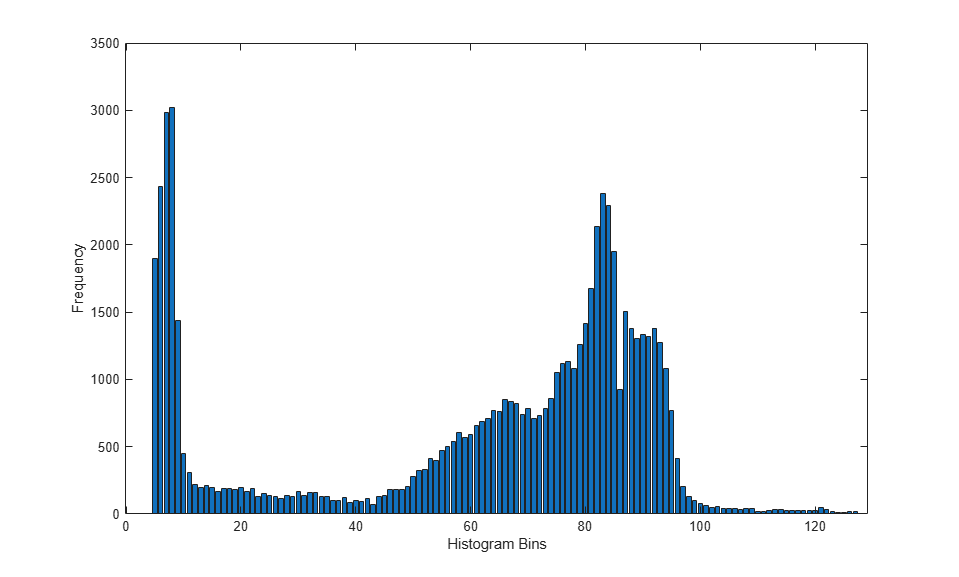# Generate Image Histogram

This example shows how to generate the histogram of an image using `2-D` `Histogram` block. The model outputs a bar plot that shows the frequency of occurrence for pixels values in the input image.

Read an input image to the MATLAB workspace.

```I = imread('cameraman.tif'); ```

Find the maximum intensity value in the input image.

```maxI = max(I(:)); ```

Open the simulink model. The model reads the image stored in variable `I` from the MATLAB workspace. The `Upper` `limit` `of` `histogram` parameter of the `2-D Histogram` block is set to the maximum value of the intensity image. The `Number` `of` `Bins` parameter of the `2-D Histogram` block is set to 128 and the histogram is computed for the entire input.

```modelname = 'ex_blkhistogram.slx'; open_system(modelname); ```The model outputs a time series that specifies the frequency of occurrence of pixels within each bin. Export the histogram values to MATLAB workspace and plot the histogram.

```out = sim(modelname); bar(out.hist.data) xlabel('Histogram Bins') ylabel('Frequency') ```